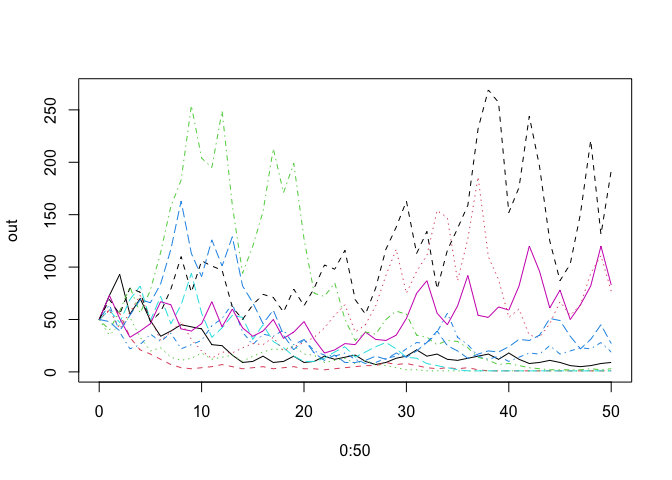# primer

The goal of primer is to .maintain functions used in [Primer of Ecology Using R] (https://hankstevens.github.io/Primer-of-Ecology/)

## Installation

You can install the released version of primer from CRAN with:

``install.packages("primer")``

And the development version from GitHub with:

``````# install.packages("devtools")
devtools::install_github("HankStevens/primer")``````

## Example

Stochastic density-independent population dynamics.

``````library(primer)

# random finite rates of increase
lambdas <- runif(10, .5, 1.5)

# stochastic dynamics
out <- PopSim(Rs=lambdas, years=50, N0=50)
matplot(0:50, out, type='l')````````````
# distribution of final population sizes
summary(out[51,])
#>    Min. 1st Qu.  Median    Mean 3rd Qu.    Max.
#>     1.0     1.5    14.0    41.3    64.5   192.0``````